Mobile QR Code1. (Department of Nanoenergy Engineering, College of Nanoscience and Nanotechnology, Pusan National University, Busan 42641, Korea)

3-D MOSFET, reconfigurable filedeffect transistor (RFET), vertically stacked gates

## I. INTRODUCTION

During the last few decades, the scaling down of metal-oxide-semiconductor field-effect transistors (MOSFETs) has been the main stream in electronics. However, scaling down, based on Moore's Law, will end in the near future after the sub-10 nm node. In this circumstance, several next-generation transistors have been investigated. Among them, reconfigurable fieldeffect transistors (RFETs) can be dynamically configured as n or p-type FET by changing electric signals during operation[1-5]. RFETs operated in the n-type mode or ptype modes can vary the Schottky barrier height of the silicon channel by applying a voltage to the gate. Through this reconfiguration concept, it is possible to implement various logic gates with fewer reconfigurable transistors than conventional complementary metaloxide-semiconductor (CMOS) technology[6-8]. For example, RFETs require only half of the transistors to construct XOR logic gates, when compared to CMOS implementation. In addition, since the RFET does not require a doping process, it can reduce fabrication steps and device variations due to the random dopant fluctuation. However, it is disadvantaged in terms of device area because it requires two or more gates.

In this paper, to overcome the drawback of RFETs, we propose a novel RFET structure with two vertical silicon nanowires connected to the bulk, and vertically stacked gates. The characteristics of the proposed device were examined through a technology computer-aided design (TCAD) simulation, using Atlas SilvacoTM V5.20.2.R.

## II. DEVICE STRUCTURE AND PROCESS

### 1. Principles of RFET Operation

The conventional RFET has two types of gates: polarity gates (PG) and control gates (CG). The CG controls the transistor $on$ and $off$ similar to the traditional gate of a MOSFET. On the other hand, the PGs near the source/drain Schottky metal contacts determine the type of the majority carrier for current conduction. A positive PG bias causes electron injection at Schottky contacts and electron current flows for the $n$-type operation, while a negative PG bias causes hole injection and hole current flows for the p-type operation. Fig. 1 shows the band energy diagram for RFET operation according to the biases of the PGs and CG.

Fig. 1. Band diagrams for the working principle of the RFET.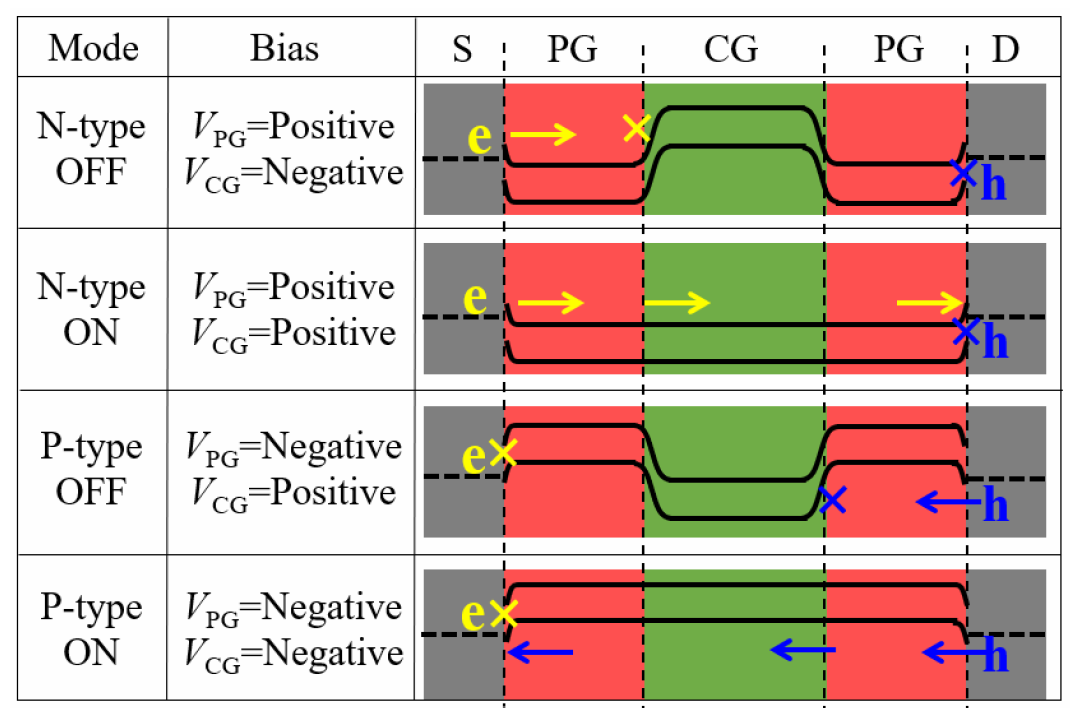This RFET device can provide an efficient circuit configuration by a fine grain reconfiguration of simple as well as complex logic functions. However, the previous RFET devices have a drawback: The unit cell size is large, due to the added gates, compared to a conventional MOSFET with only one gate. To solve this problem, we proposed a U-shaped RFET.

### 2. U-shaped RFET Structure

Fig. 2(a) indicates the conventional RFET structure. Although the two PGs are applied to the same operation voltage, they are physically separated. To reduce the area burden due to the PGs, we revised the structure as shown in Fig. 2(b). The two PGs are combined into one by folding the channel. Thus, the 3-D structure of the Ushaped RFET was proposed as shown in Fig. 2(c). The proposed U-shaped RFET has several advantages over previous RFETs. First, the device size can be reduced by combining two PGs together and by stacking the CG and PG vertically as illustrated in Fig. 2(c). Second, it exhibits excellent scalability without any degradation of the subthreshold swing (SS) or drain-induced barrier lowering (DIBL), since its main channel length is defined vertically.

Fig. 2. (a) Conventional RFET structure, (b) Conceptual diagram of the proposed device, (c) U-shaped RFET structure and cell size comparison.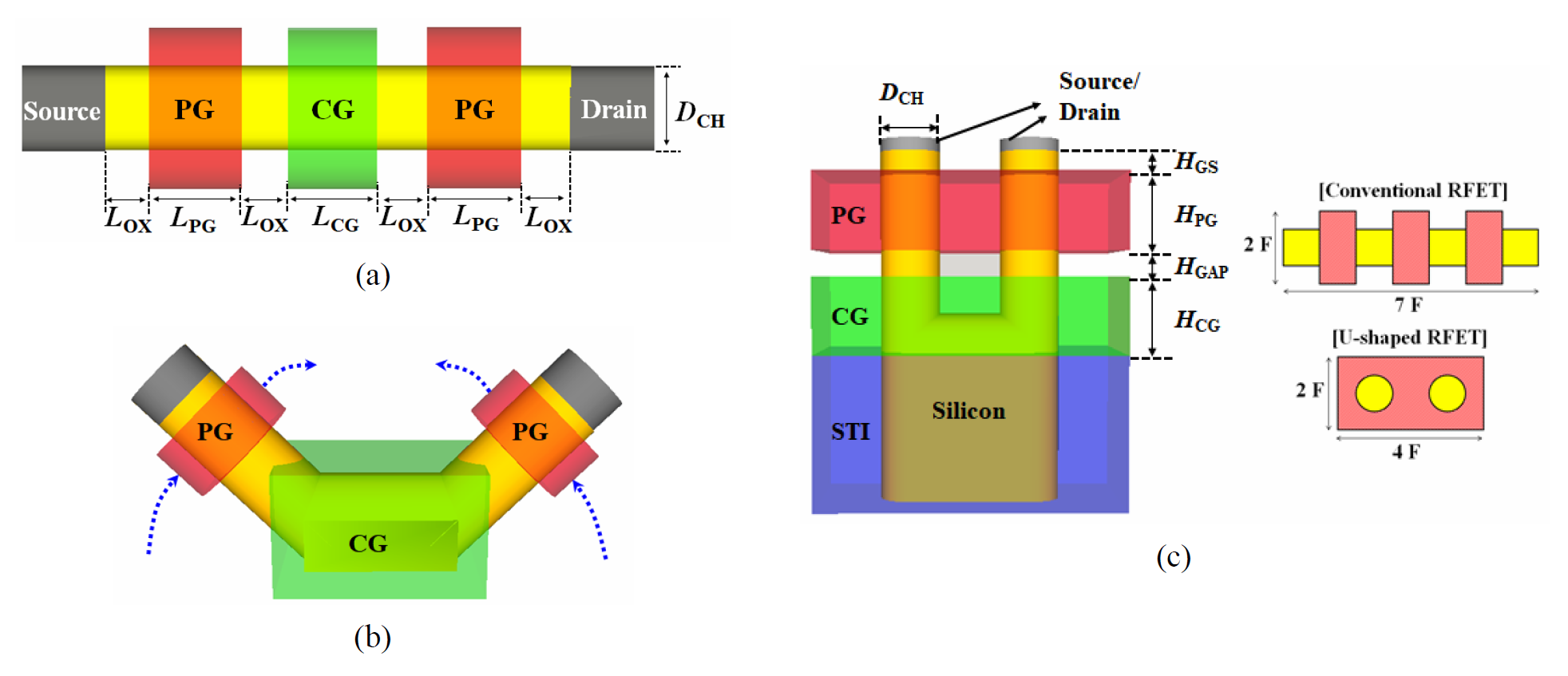To verify the characteristics of the proposed device, a TCAD simulation was conducted. The device parameters used for simulation are summarized in Table 1. In addition, thermionic emission model, barrier lowering model, and barrier tunneling models (Wentzel-Kramers- Brillouin approximation) are used for the carrier injection through source/drain Schottky contacts. Electron and hole tunneling masses are chosen as 0.19 and 0.16 $m_{0}$ (the electron rest mass), respectively, which is consistent with the literature values.

Table 1. Parameters of the baseline of designed device

 Gate metal work-function($W_{G}$) 4.5 eV Source/Drain metal work-function ($W_{SD}$) 4.75 eV CG height ($H_{CG}$) 15 nm PG height ($H_{PG}$) 15 nm Channel Diameter ($D_{CH}$) 10 nm Gap between Source/Drain and PG ($H_{GS}$) 5 nm CG to PG height ($H_{GAP}$) 5 nm Equivalent gate oxide thickness 2 nm Silicon doping concentration (Boron) 1 × 1015 cm-3 Conventional CG length ($L_{CG}$) 15 nm Conventional PG length ($L_{PG}$) 15 nm Conventional oxide length ($L_{OX}$) 5 nm Source/Drain voltage 0.7 V PG Voltage in N-type mode 2.0 V PG Voltage in P-type mode -2.0 V

Fig. 3 shows a comparison of transfer characteristics between conventional RFET and U-shaped RFET. A turn-on voltage ($V_{turn-on}$) is defined as the CG voltage ($V_{CG}$) when the drain current ($I_{d}$) is 10-12 A. An average SS ($SS_{avg}$) indicates a reciprocal of mean ratio of change in the log($I_{D}$) -$V_{G}$ curve, when $I_{d}$ increases from 10-12 A to 10-7 A. Lastly, $I_{on}$ is extracted for $V_{CG}$ = $Vturn-on}$ + 0.7 V.

Fig. 3. Transfer characteristics of a conventional RFET and the U-shaped RFET.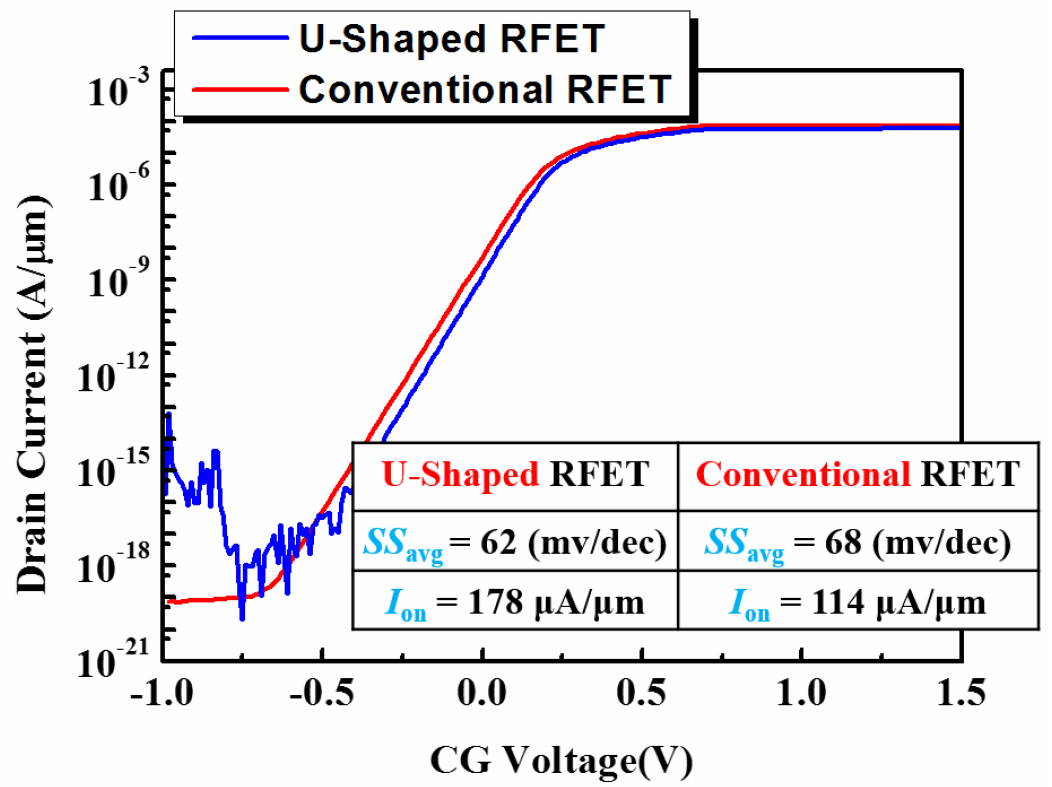As indicated in Fig. 3, similar characteristics to the conventional RFET can be achieved. Both U-shaped and conventional RFETs exhibit SS values below 70 mV/dec, which are attributed to the good gate controllability of Gate-All-Around (GAA) structure.

### 3. Fabrication Process

Fig. 4 illustrates the critical fabrication steps of the designed U-shaped RFET. The specific method of each step is summarized as follows. (a) First, active channel regions are formed using the silicon nitride hard mask, which will be used as the chemical mechanical polishing (CMP) stopper in the later planarization steps. (b) Oxide sidewall along the vertical channel is formed by the sequential deposition and etching process. (c) By using oxide sidewall as a hard mask, silicon etching is carried out. (d) Selective silicon wet etching is carried out. Consequently, the U-shaped channel region is formed on the substrate. (e) The oxide sidewall is removed by the selective wet etching. In addition, a silicon corner rounding process is performed. (f) Oxide deposition, CMP, and etch-back are carried out to form the shallow trench isolation (STI) region. (g) Gate dielectric (under the CG region) and the CG are formed. The formation of the CG is carried out by the deposition, CMP, and etchback steps. (h) Gate dielectric (under the PG region) is deposited. At this time, this gate dielectric layer is also used for the isolation between CG and PG. Then, the formation of PG occurs by the deposition, CMP, and etch-back steps. (i) Finally, the source and drain contact process is carried out. In the exposed silicon region, which is formed by the selective removal of silicon nitride, the self-aligned silicide process can be used.

## III. DEVICE CHARACTERISTICS STUDY

In this chapter, the effects of various device parameters such as the gap between source/drain and PG ($H_{GS}$), work-function of the source/drain materials ($W_{SD}$), and channel diameter ($D_{CH}$). The default parameters used in this study are listed in Table 1.

First, the dependency of device performance on the $H_{GS}$ was simulated. As illustrated in Fig. 5, when $H_{GS}$ has a larger positive value (which means the underlap between source/drain and $PG_{s}$), the degradation of $I_{on}$ occurs. As the gap between the source/drain and PG increases, the electric field at the source/drain junction decreases, resulting in Schottky tunneling decrease. As a result, the source/drain structure overlapped with the PG is required to obtain high current drivability.

Fig. 5. Transfer characteristics with the variation of $H_{GS}$.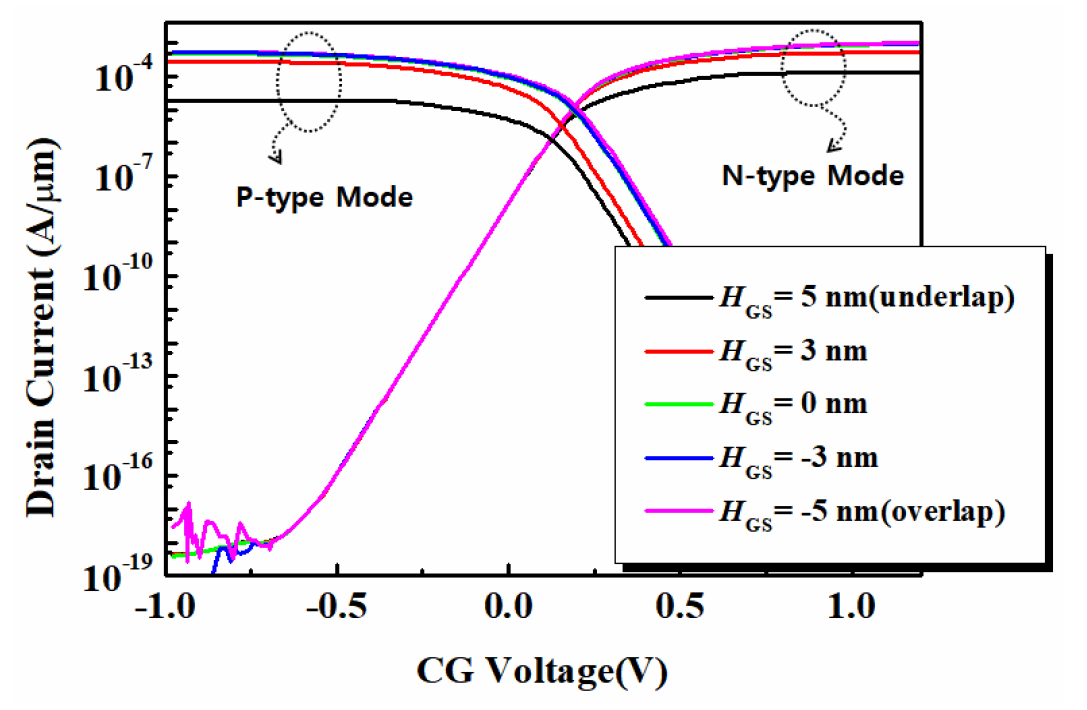Second, the influence of source/drain metal work function ($W_{SD}$) was studied. Fig. 6 shows the transfer characteristics of $n$-type and $p$-type operations depending on $W_{SD}$. Since $I_{ON}$ of RFETs is dominated by Schottky contact resistance at source/drain junction, which is generally much larger than channel resistance, as is well known, the Schottky barrier height is a dominant factor for current drivability of RFETs. When the Fermi level of the source/drain metal is close to the intrinsic Fermi level of the silicon ($W_{\mathrm{SD}} \approx 4.71 \mathrm{eV}$), symmetrical characteristics between the $n$-type and $p$-type modes can be obtained. The Fermi-level modulation of the nickel silicide can be achieved by controlling the content of nickel or aluminum ion implantation process. If $W_{SD}$ is relatively small, the Schottky barrier height for electron injection is smaller than that of hole injection, resulting in $I_{on,N}$ > $I_{on,P}$ and vice versa.

Third, the dependency of device characteristics on the channel diameter ($D_{CH}$) was investigated as illustrated in Fig. 7. As the $D_{CH}$ decreases, the $SS_{avg}$ value becomes smaller due to the maximization of the electric field concentration effect of the GAA geometry.

Fig. 7. Transfer characteristics with the variation of $D_{CH}$.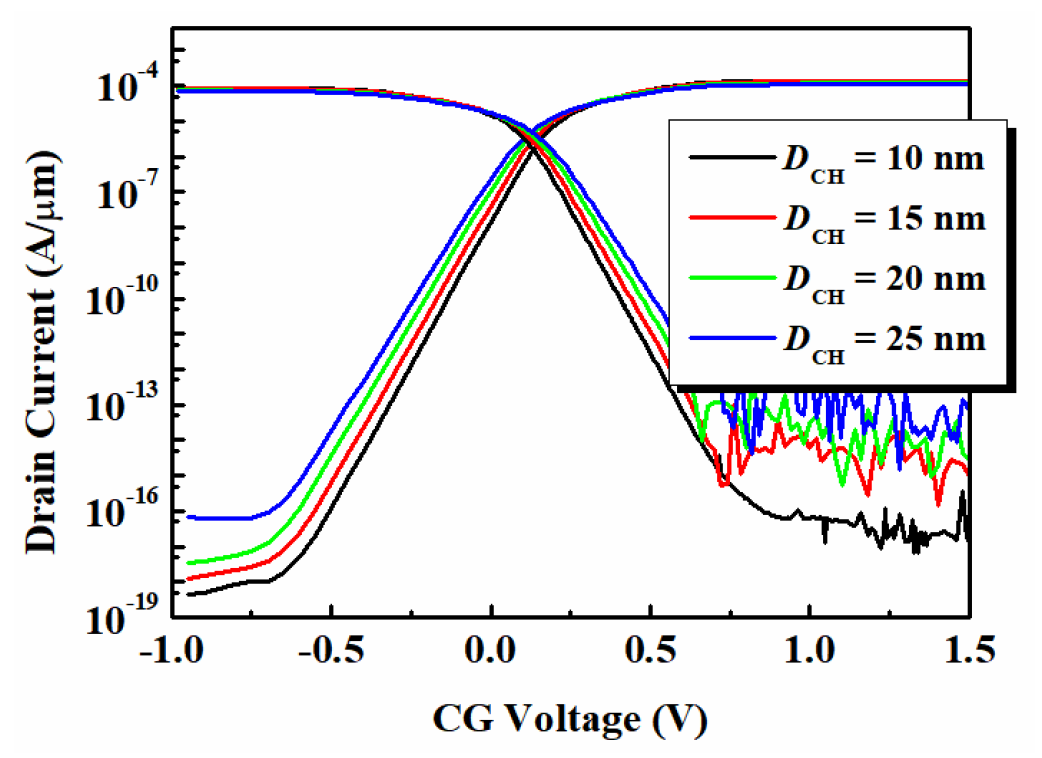## IV. SUMMARY

In this paper, we have proposed a novel U-shaped RFET which has vertically stacked gates and a U-shaped nanowire channel. Through this unique device structure, the size of the proposed U-shaped RFET device can be reduced by about 40% compared to conventional RFETs. Using a TCAD simulation, we successfully demonstrated the reconfigurable operation of the proposed device. For the device optimization, the effects of various device parameters on the device performance were also investigated. To ensure good current drivability, there must be an overlap between the source/drain region and the PG so that sufficient amount of carrier injection can occur at the Schottky junction. Also, the symmetry of n and p-modes can be obtained by adjusting the work function of the source/drain material. It is expected that the proposed U-shaped RFET will be one of the most promising candidate for a next-generation transistor.

### ACKNOWLEDGMENTS

This research was supported by Basic Science Research Program through the National Research Foundation of Korea(NRF) funded by the Ministry of Science, ICT & Future Planning(2016R1C1B2013257)

### REFERENCES

1
De Marchi M., Sacchetto D., Frache S., Zhang J., Gaillardon P. E., Leblebici Y., De Micheli G., 2012, Polarity control in double-gate, gate-all-around vertically stacked silicon nanowire FETs, Proc. IEDM, pp. 8.4.1-8.4.42
Heinzig A., Slesazeck S., Kreupl F., Mikolajick T., Weber W. M., Jan. 2012, Reconfigurable silicon nanowire transistors, Nano Letters, Vol. 12, No. 1, pp. 119-1243
Gaillardon Pierre-Emmanuel, Gaetano Amarù Luca, Bobba Shashikanth, De Marchi Michele, Sacchetto Davide, Leblebici Yusuf, De Micheli Giovanni, Apr. 2004, Vertically-Stacked Double-Gate Nanowire FETs with Controllable Polarity: From Devices to Regular ASICs, Solid-State Circuits, IEEE Journal of, Vol. 39, No. 4, pp. 602-6124
Zhang J., Marchi M. D., Sacchetto D., Gaillardon P. E., Leblebici Y., Micheli G. D., Oct. 2014, Polarity-Controllable Silicon Nanowire Transitors With Dual Threshold Voltages, Electron Devices, IEEE Transacions on, Vol. 61, No. 11, pp. 3654-36605
Gaillardon P. E., Hasan M., Saha A., Amaru L., Walker R., Rodriquez B. S., 2016, Digital, analog and RF design opportunities of three-dindependent-gate transistors, Proc. ISCAS, pp. 405-4086
Zhang J., Tang X., Gaillardon P. E., De Micheli G., Oct. 2014, Configurable circuits featuring dualthreshold-voltage design with three-independentgate silicon nanowire FETs, Circuits Syst. I. Reg. Papers, IEEE Transactions on, Vol. 61, No. 10, pp. 2581-28617
Trommer J., Heinzig A., Slesazeck S., Mikolajick T., Weber W. M., Jan. 2014, Elementary aspects for circuit implementation of reconfigurable nanowire transistors, Electron Device Lett., IEEE, Vol. 35, No. 1, pp. 141-1438
De Marchi M., Zhang J., Frache S., Sacchetto D., Gaillardon P. E., LEblebici Y., Micheli G. D., Aug. 2014, Configurable logic gates using polarity-controlled silicon naowire gate-all-around FETs, Electron Device Lett., IEEE, Vol. 35, No. 8, pp. 880-8829
Bhattacharjee A., Dasqupta S., Mar. 2016, Optimization of Design Parameters in Dual-$k$ Spacer-Based Nanoscale Reconfigurable FET for Improved Performance, Electron Devices, IEEE Transacions on, Vol. 63, No. 3, pp. 1375-138210
Tsuchiya Y., Yoshiki M., Koga J., Nishiyama A., Koyama M., Aug. 2009, Influence of Ni silicide phases on effective work function modulation with Alpileup in the Ni fully silicided gate/HfSiON system, Journal of Applied Physics, Vol. 106, pp. 04451011
Singh N., Agarwal A., Bera L. K., Liow T. Y., Yang R., Rustagi S. C., Tung C. H., Kumar R., Lo G. Q., Balasubramanian N., Kwong D. L., Bhattacharjee A., Dasqupta S., May. 2006, High-Performance Fully Depleted Silicon Nanowire (Diameter ≤ 5 nm) Gate-All-Around CMOS Devices, Electron Device Lett., IEEE, Vol. 27, No. 5, pp. 383-386## Author

received a B.S. degree in nanomaterials engineering from Pusan National University, Busan, South Korea in 2017, where he is currently pusuing an M.S. degree.

received a B.S. degree in nanomaterials engineering from Pusan National University, Busan, South Korea in 2017, where he is currently pusuing an M.S. degree.

received a B.S. and M.S. degrees in nanomaterials engineering from Pusan National University, Busan, South Korea in 2016 and 2018, respectively.

received a B.S. degree in nanomaterials engineering from Pusan National University, Busan, South Korea in 2017, where he is currently pusuing an M.S. degree.

received a B.S. degree in nanomaterials engineering from Pusan National University, Busan, South Korea in 2017, where he is currently pusuing an M.S. degree.

received a B.S. degree in nanomaterials engineering from Pusan National University, Busan, Korea, in 2018, where he is currently pursuing an M.S. degree.

(M’15) received B.S. and Ph.D. degrees in electrical engineering from Seoul National University, Seoul South Korea, in 2006 and 2012, respectively.

From 2012 to 2015, he was a Senior Engineer with Samsung Electronics Company, Suwon, South Korea.

In 2015, he joined Pusan National University, Busan, South Korea, as an Assistant Professor.